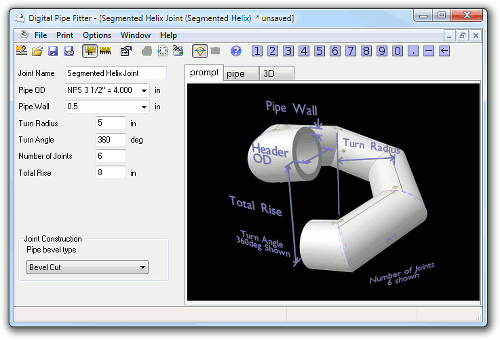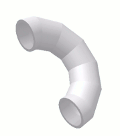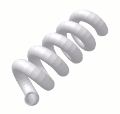# Segmented Helix Jointback to other joints

A Segmented Helix Joint is a way to create a spring shaped structure using multiple segments of straight pipe.

A single template is generated that can be used to cut each segment. The first and last segments can be any length, so only use one cut line for these segments.

## Parameter Constraints:

Joint Name:  name of this joint which is unique to this project or currently opened joints

Pipe OD: (Outside Diameter) must be a positive number

Pipe Wall: (Wall Thickness) must be a positive number, less than half Pipe OD

Turn Angle: and positive number (yes it can be more than 360 deg.) Use a negative Total Rise to create a counter-clockwise spiral.

Number of Joints: The number of joints used to create the curve. A positive number.

Total Rise: distance between openings. Note that because of the rise angle, the distance between the two pipes feeding into and out of the helix is not equal to the Total Rise. The Total Rise is not the measurement between the two parallel pipes at the input and output but between the openings themselves.
For a valid joint with Turn Angle greater than 360 deg., the Total Rise must be large enough so that the next spiral of pipe does not interfere with the previous spiral.
A negative value for Total Rise will produce a counter-clockwise Helix.

## Creative Uses:

 When Total Rise is 0, this joint is identical to the Segmented Turn Joint. Total Rise=0Turn Angle=180A coil is generated if Turn Angle is greater than 360 deg. Turn Angle = 1800Same as above but with fewer Number of Joints. Turn Angle = 1800Number of Joints = 15Just for fun. This is an invalid joint because the pipes interfere with each other. But it's pretty to look at. Have fun! Pipe OD=5Turn Radius=9 Turn Angle=2880Number of Joints = 19Total Rise=0This joint is of very little practical use. I guess it could be used to build a spiral staircase.